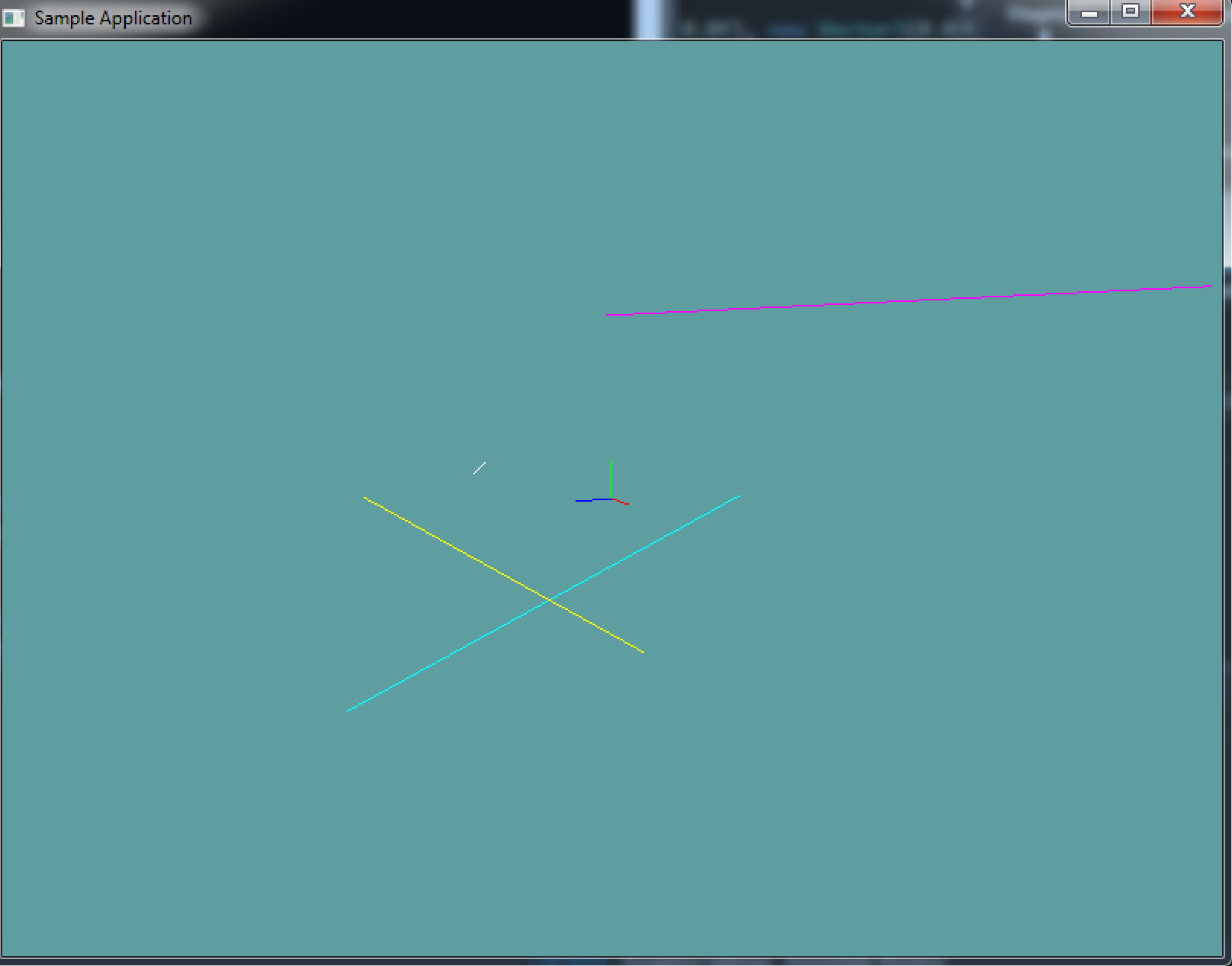# Line

Technically a line is the intersection of 2 planes. It runs infinatley in all directions. What we're going to calla line is actually a Line Segment. It's a line between two points. Unlike a line, a line segment does have a length.

Given the above definition, defining a line is pretty simple:

class Line {
public Point start;
public Point end;
}


There is really no magic to a line. For example if you want to get the length of a line, it's the same as getting the length of a vector! You get a vector by subtracting start from end, then find the length!

### Code Guide

The Line class is straight forward. You can follow this guide for implementation, or make your own.

using System;
using OpenTK.Graphics.OpenGL;
using Math_Implementation;

namespace CollisionDetectionSelector.Primitives {
class Line {
public Point start = new Point();
public Point end = new Point();

public float Length { get { /*TODO*/ } }
public float LengthSquared { get { /*TODO*/ } }

public Line() {
// Make a line of 0 length,
// both points should default to (0, 0, 0)
}

public Line(Line other) {
// TODO: Copy the other line
// Pay attention, are you assigning by reference or value
}

public Line(Point p1, Point p2) {
// TODO
}

public Line(Vector3 p1, Vector3 p2) {
// TODO
}

public Vector3 ToVector() {
// TODO: return end - start
}

//Render and toString must be there!
public void Render() {
GL.Begin(PrimitiveType.Lines);
GL.Vertex3(start.X, start.Y, start.Z);
GL.Vertex3(end.X, end.Y, end.Z);
GL.End();
}

public override string ToString() {
string result = "Start: (" + start.X + ", " + start.Y + ", " + start.Z + "), ";
result += "End: ( " + end.X + ", " + end.Y + ", " + end.Z + ")";
return result;
}
}
}


Implement the Line class. You can use the code guide above, or use your own implementation

### Sample / Unit Test

You can Download the samples for this chapter to see if your result looks like the unit test.

This example is visual only, no errors will be printed to the console if the code is bad. This is what the final render should look like:using OpenTK.Graphics.OpenGL;
using Math_Implementation;
using CollisionDetectionSelector.Primitives;

namespace CollisionDetectionSelector.Samples {
class LineSample : Application {
protected Vector3 cameraAngle = new Vector3(120.0f, -10f, 20.0f);
protected float rads = (float)(System.Math.PI / 180.0f);

Line[] origin = new Line[] {
new Line(new Point(0, 0, 0), new Point(1, 0, 0)),
new Line(new Point(0, 0, 0), new Point(0, 1, 0)),
new Line(new Point(0, 0, 0), new Point(0, 0, 1))
};

Line[] random = new Line[] {
new Line(new Point(-7, 5, -3), new Point(8, 5, -10)),
new Line(new Point(-8, -8, 6), new Point(-9, -2, -10)),
new Line(new Point(-4, -6, -3), new Point(-9, -1, 5)),
new Line(new Point(-6, 0, 2), new Point(-1, 1, 3))
};

float[][] colors = new float[][] {
new float[] { 1f, 0f, 1f },
new float[] { 0f, 1f, 1f },
new float[] { 1f, 1f, 0f },
new float[] { 1f, 1f, 1f },
};

public override void Intialize(int width, int height) {
GL.Enable(EnableCap.DepthTest);

/*System.Random r = new System.Random();
string output = "new Line(new Point(" + (r.Next() % 20 - 10) + ", " + (r.Next() % 20 - 10) + ", " + (r.Next() % 20 - 10) + "), new Point(" + (r.Next() % 20 - 10) + ", " + (r.Next() % 20 - 10) + ", " + (r.Next() % 20 - 10) + ")),\n";
for (int i = 0; i < 3; ++i) {
output += "new Line(new Point(" + (r.Next() % 20 - 10) + ", " + (r.Next() % 20 - 10) + ", " + (r.Next() % 20 - 10) + "), new Point(" + (r.Next() % 20 - 10) + ", " + (r.Next() % 20 - 10) + ", " + (r.Next() % 20 - 10) + ")),\n";
}
System.IO.File.WriteAllText(@"C:\Users\WinVPC\Desktop\Lines.txt", output);*/
}

public override void Render() {
Vector3 eyePos = new Vector3();
eyePos.Y = cameraAngle.Z * -(float)System.Math.Sin(cameraAngle.Y * rads);

Matrix4 lookAt = Matrix4.LookAt(eyePos, new Vector3(0.0f, 0.0f, 0.0f), new Vector3(0.0f, 1.0f, 0.0f));

GL.Color3(1f, 0f, 0f);
origin.Render();
GL.Color3(0f, 1f, 0f);
origin.Render();
GL.Color3(0f, 0f, 1f);
origin.Render();

for (int i = 0; i < random.Length; ++i) {
GL.Color3(colors[i], colors[i], colors[i]);
random[i].Render();
}
}

public override void Update(float deltaTime) {
cameraAngle.X += 45.0f * deltaTime;
}

public override void Resize(int width, int height) {
GL.Viewport(0, 0, width, height);
GL.MatrixMode(MatrixMode.Projection);
float aspect = (float)width / (float)height;
Matrix4 perspective = Matrix4.Perspective(60, aspect, 0.01f, 1000.0f);